# The Stefan-Boltzmann Law

The total power per unit area from a blackbody radiator can be obtained by integrating the Planck radiation formula over all wavelengths. The radiated power per unit area as a function of wavelength is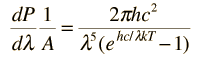so the integrated power is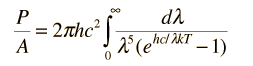It is helpful to make the substitution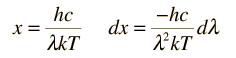Making the substitution gives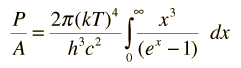Making use of the standard form integral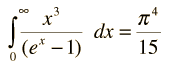gives the final form of the Stefan-Boltzmann law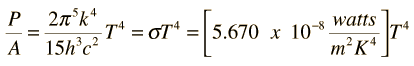Calculation of radiated power
Index